# How can I replicate Quantum Break's distortion particle effect?

Quantum Break has this fantastic particle effect, it's a distortion effect like broken glass. I want know how I can replicate this effect? You can see it below, and a full video is available on YouTube: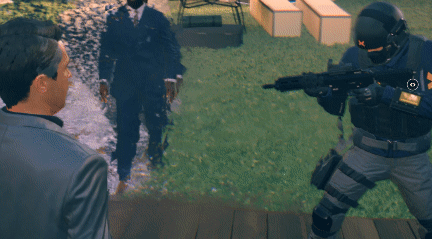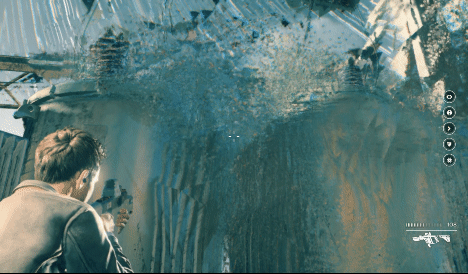• "This question has not received enough attention."? – Vaillancourt Feb 7 '18 at 17:31
• @AlexandreVaillancourt I just pin this question for more views and draw attention because I think it's useful.I couldn't find better reason on bounty reasons If there is a problem I will change my reason.thanks – Seyed Morteza Kamali Feb 7 '18 at 18:01
• The purpose of bounties isn't really just to "pin" questions though; your stated rationale is somewhat disingenuous. The purpose of bounties is to drawn attention to questions that need answers or to reward existing answers, which yours isn't doing. There are already mechanisms in place (the HNQ, which many of your posts hit) to reward topics the community feels are useful and interesting. – user1430 Feb 7 '18 at 19:40
• Dont be greedy. You already got enough views and votes – Casanova Feb 7 '18 at 21:05
• @JoshPetrie you're right I'm sorry I don't repeat this state.I'm Ashamed so you can remove my question from features without returning reputation.I just do this because I thought Maybe I will help others. – Seyed Morteza Kamali Feb 8 '18 at 3:45

# Pyramid Particle

Unity default particle's shape is quad. first you need to change this shape to pyramid by using pyramid object or turns quads to pyramids with a geometry shader.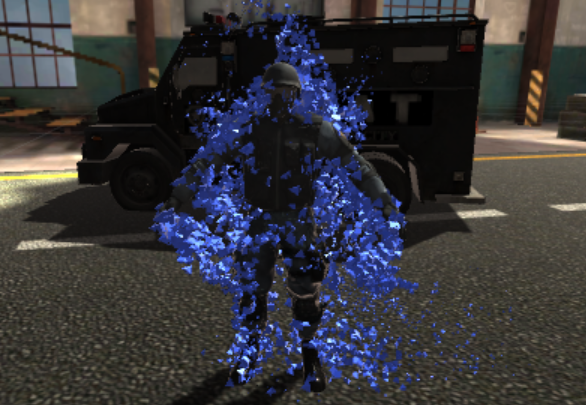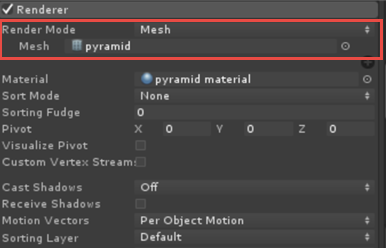# Refraction

To making broken glass effect(Refraction) you can use GrabPass { "TextureName" } that will grab screen contents into a texture.

GrabPass is a special pass type - it grabs the contents of the screen where the object is about to be drawn into a texture. This texture can be used in subsequent passes to do advanced image based effects.

https://docs.unity3d.com/Manual/SL-GrabPass.html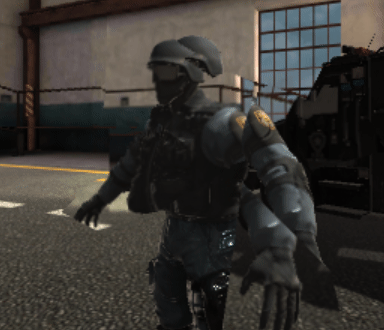Shader "Smkgames/GlassRefraction"
{
Properties{
_Refraction("Refraction",Float) = 0.05
_Alpha("Alpha",Range(0,1)) = 1
}
{
Tags {"Queue"="Transparent" "RenderType"="Transparent"}

Blend SrcAlpha OneMinusSrcAlpha

GrabPass
{
"_GrabTexture"
}

Pass
{
CGPROGRAM
#pragma vertex vert
#pragma fragment frag

#include "UnityCG.cginc"

struct appdata
{
float4 vertex : POSITION;
};

struct v2f
{
float4 grabPos : TEXCOORD0;
float4 vertex : SV_POSITION;
};
sampler2D _MainTex;
float _Alpha,_Refraction;

v2f vert (appdata v)
{
v2f o;
o.vertex = UnityObjectToClipPos(v.vertex);
o.grabPos = ComputeGrabScreenPos(o.vertex);
return o;
}

sampler2D _GrabTexture;

fixed4 frag (v2f i) : SV_Target
{
fixed4 col = tex2Dproj(_GrabTexture, i.grabPos+_Refraction);
return float4(col.rgb,_Alpha);

}
ENDCG
}
}
}


# Using mesh normals

Let’s proceed with a shader that displays mesh normals in world space. I used it because I wanted to look three dimensional broken shape.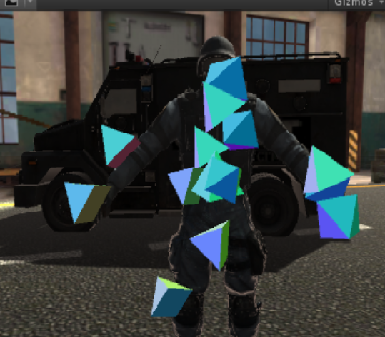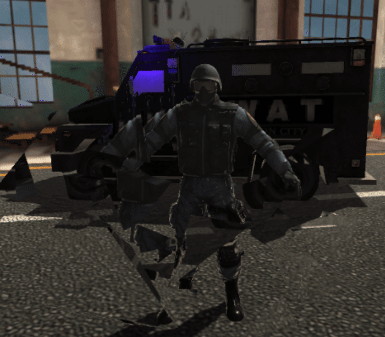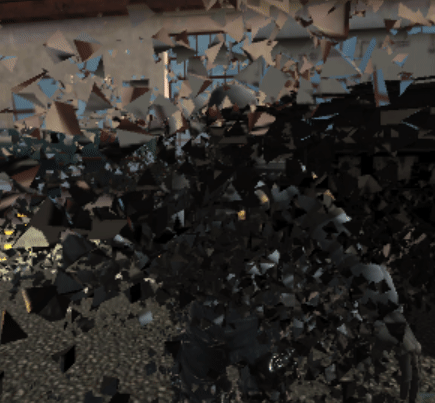Shader "Smkgames/BrokenGlass3D"
{
Properties{
_MainTex("MainTex",2D) = "white"{}
_Alpha("Alpha",Float) = 1
}
{
Tags {"Queue"="Transparent" "RenderType"="Transparent"}
Blend SrcAlpha OneMinusSrcAlpha

GrabPass
{
"_GrabTexture"
}

Pass
{
CGPROGRAM
#pragma vertex vert
#pragma fragment frag

#include "UnityCG.cginc"

struct appdata
{
float4 vertex : POSITION;
float2 grabPos : TEXCOORD0;
float3 normal :NORMAL;
};

struct v2f
{
float4 grabPos : TEXCOORD0;
float4 vertex : SV_POSITION;
half3 worldNormal :TEXCOORD1;

};
sampler2D _MainTex;
float _Intensity,_Alpha;

v2f vert (appdata v)
{
v2f o;
o.vertex = UnityObjectToClipPos(v.vertex);
o.grabPos = ComputeGrabScreenPos(o.vertex);
o.worldNormal = UnityObjectToWorldNormal(v.normal);
return o;
}

sampler2D _GrabTexture;

fixed4 frag (v2f i) : SV_Target
{
fixed4 c = 0;
c.rgb = i.worldNormal*0.5+0.5;
float4 distortion = tex2D(_MainTex,i.grabPos)+_Intensity;
fixed4 col = tex2Dproj(_GrabTexture, i.grabPos+c.r);
return float4(col.rgb,_Alpha);
}
ENDCG
}
}
}


# Heat Distortion

To creating heat distortion you can use flow map

A flow-map is a texture that stores 2d directional information in a texture. The color of the pixel determines what direction it is using the uv-coordinates texture as a base. The more color there is the faster the proportional speed. Example green tells it to go up-left, the center is neutral, and red will go down right. It’s a useful technique for liquid materials like water, and a useful alternative to just a panner node.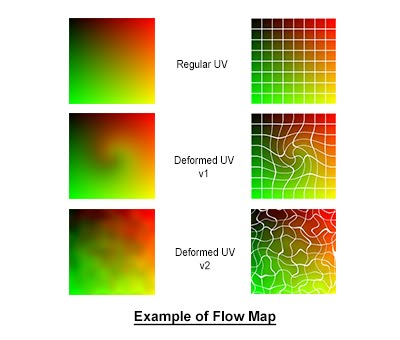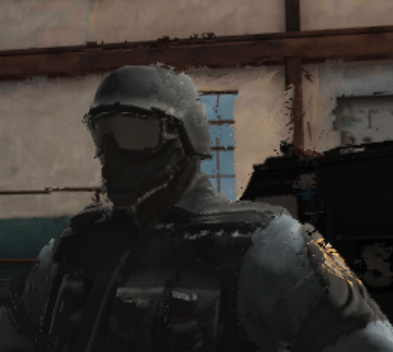Shader "Smkgames/HeatDistortion"
{
Properties{
_DistortionMap("DistortionMap",2D) = "white"{}
_Intensity("Intensity",Float) = 50
_Alpha("Alpha",Range(0,1)) = 1
}
{
Tags {"Queue"="Transparent" "RenderType"="Transparent"}

GrabPass
{
"_GrabTexture"
}

Blend SrcAlpha OneMinusSrcAlpha

Pass
{
CGPROGRAM
#pragma vertex vert
#pragma fragment frag

#include "UnityCG.cginc"

struct appdata
{
float4 vertex : POSITION;
};

struct v2f
{
float4 grabPos : TEXCOORD0;
float4 vertex : SV_POSITION;
};
float _Alpha,_Refraction;

v2f vert (appdata v)
{
v2f o;
o.vertex = UnityObjectToClipPos(v.vertex);
o.grabPos = ComputeGrabScreenPos(o.vertex);
return o;
}

sampler2D _GrabTexture;
float _Intensity;

fixed4 frag (v2f i) : SV_Target
{
float4 distortion = tex2D(_DistortionMap,i.grabPos+_Time.y)+_Intensity;
fixed4 col = tex2Dproj(_GrabTexture, i.grabPos*distortion);

}
ENDCG
}
}
}


another example by using normal: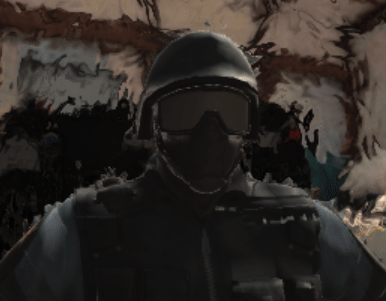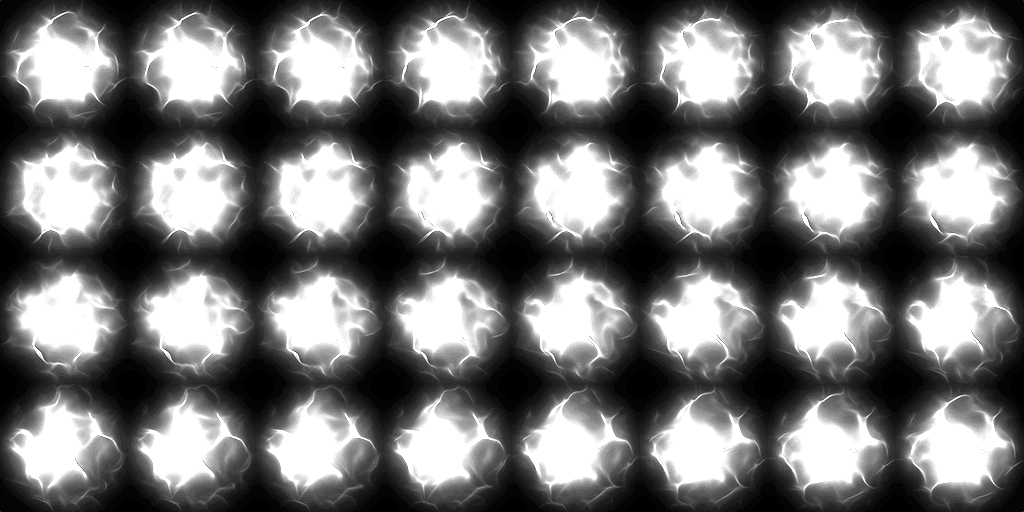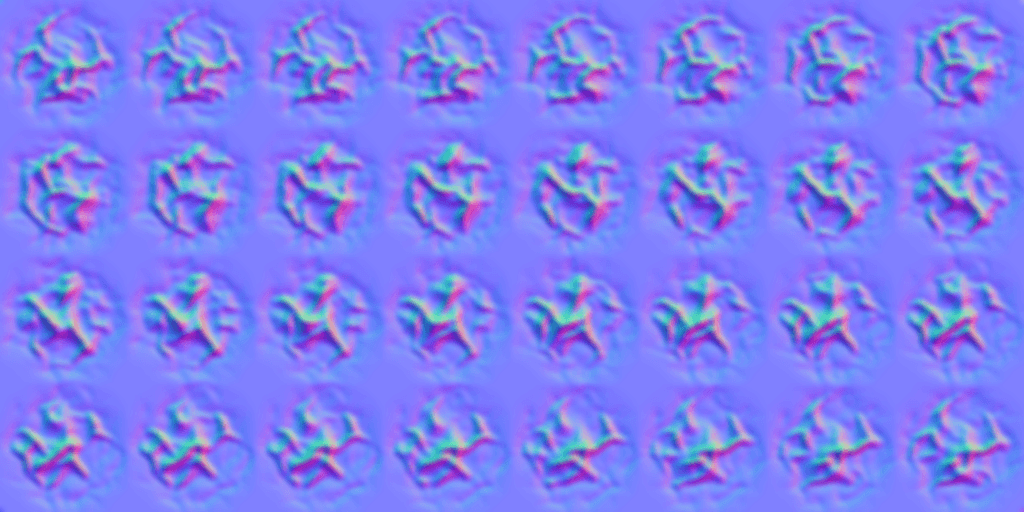Shader "Smkgames/HeatDistortion2" {
Properties {
_CutOut ("CutOut (A)", 2D) = "black" {}
_BumpMap ("Normalmap", 2D) = "bump" {}
_BumpAmt ("Distortion", Float) = 10
}

Category {

Tags { "Queue"="Transparent"  "IgnoreProjector"="True"  "RenderType"="Opaque" }
Blend SrcAlpha OneMinusSrcAlpha
Cull Off
Lighting Off
ZWrite Off
Fog { Mode Off}

GrabPass {
"_GrabTexture"
}
Pass {

CGPROGRAM
#pragma vertex vert
#pragma fragment frag
#pragma fragmentoption ARB_precision_hint_fastest
#pragma multi_compile_particles
#include "UnityCG.cginc"

struct appdata_t {
float4 vertex : POSITION;
float2 texcoord: TEXCOORD0;
};

struct v2f {
float4 vertex : POSITION;
float4 uvgrab : TEXCOORD0;
float2 uvbump : TEXCOORD1;
float2 uvcutout : TEXCOORD2;
};

sampler2D _BumpMap,_CutOut,_GrabTexture;
float _BumpAmt;
float4 _GrabTexture_TexelSize;
float4 _BumpMap_ST,_CutOut_ST;

v2f vert (appdata_t v)
{
v2f o;
o.vertex = UnityObjectToClipPos(v.vertex);
o.uvgrab.xy = (float2(o.vertex.x, o.vertex.y*-1) + o.vertex.w) * 0.5;
o.uvgrab.zw = o.vertex.zw;
o.uvbump = TRANSFORM_TEX( v.texcoord, _BumpMap );
o.uvcutout = TRANSFORM_TEX( v.texcoord, _CutOut );
return o;
}

half4 frag( v2f i ) : COLOR
{
half2 bump = UnpackNormal(tex2D( _BumpMap, i.uvbump )).rg;
float2 offset = bump * _BumpAmt * _GrabTexture_TexelSize.xy;
i.uvgrab.xy = offset * i.uvgrab.z + i.uvgrab.xy;

half4 col = tex2Dproj( _GrabTexture, UNITY_PROJ_COORD(i.uvgrab));
fixed4 cut = tex2D(_CutOut, i.uvcutout);
fixed4 emission = col;
emission.a = (cut.a);
return emission;
}
ENDCG
}
}

}
}


# RGB Split

If you pay attention to your first gif you can see little RGB split.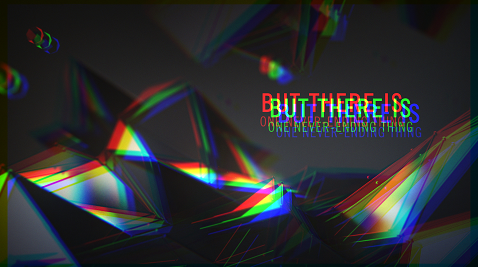Shader "Hidden/RgbSplit"
{
Properties
{
_MainTex ("Texture", 2D) = "white" {}
_NoiseTex1 ("Noise Texture A", 2D) = "white" {}
_NoiseTex2 ("Noise Texture B", 2D) = "white" {}
}
{

Pass
{
CGPROGRAM
#pragma vertex vert
#pragma fragment frag

#include "UnityCG.cginc"

struct appdata
{
float4 vertex : POSITION;
float2 uv : TEXCOORD0;
};

struct v2f
{
float2 uv : TEXCOORD0;
float4 vertex : SV_POSITION;
};

v2f vert (appdata v)
{
v2f o;
o.vertex = UnityObjectToClipPos(v.vertex);
o.uv = v.uv;
return o;
}

sampler2D _MainTex,_NoiseTex1,_NoiseTex2;
float3 colorSplit(float2 uv, float2 s)
{
float3 color;
color.r = tex2D(_MainTex, uv - s).r;
color.g = tex2D(_MainTex, uv    ).g;
color.b = tex2D(_MainTex, uv + s).b;
return color;
}

float2 interlace(float2 uv, float s)
{
uv.x += s * (4.0 * frac((uv.y) / 2.0) - 1.0);
return uv;
}

fixed4 frag (v2f i) : SV_Target
{

float t = _Time.y;

float s = tex2D(_NoiseTex1, float2(t * 0.2, 0.5)).r;

i.uv = interlace(i.uv, s * 0.005);
float r = tex2D(_NoiseTex2, float2(t, 0.0)).x;

float3 color = colorSplit(i.uv, float2(s * 0.02, 0.0));

return float4(color, 1.0);

}
ENDCG
}
}
}